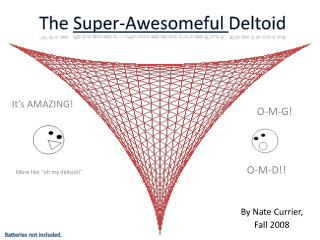DownloadDownload PresentationThe Super-Awesomeful Deltoid

# The Super-Awesomeful Deltoid

Télécharger la présentation## The Super-Awesomeful Deltoid

- - - - - - - - - - - - - - - - - - - - - - - - - - - E N D - - - - - - - - - - - - - - - - - - - - - - - - - - -
##### Presentation Transcript

1. The Super-Awesomeful Deltoid It’s AMAZING! O-M-G! By Nate Currier, Fall 2008 O-M-D!! More like “oh my deltoid!” Batteries not included.

2. Table of Contents • A brief history • The Hypocycloid • Parametric Equations • The Deltoid in ACTION! • Deltoid Description • The Deltoid in nature • The Deltoid and Man • Works Cited

3. A brief History • The deltoid has no real discoverer. • The deltoid is a special case of a Cycloid; a three-cusped Hypocycloid. • Also called the tricuspid. - It was named the deltoid because of its resemblance to the Greek letter Delta. • Despite this, Leonhard Euler was the first to claim credit for investigating the deltoid in 1754. • Though, Jakob Steiner was the first to study the deltoid in depth in 1856. • - From this, the deltoid is often known as Steiner’s Hypocycloid. Leonhard Euler, 1701-1783 Jakob Steiner, 1796-1863

4. The Hypocycloid • To understand the deltoid, aka the tricuspid hypocycloid, we must first look to the hypocycloid. • A hypocycloid is the trace of a point on a small circle drawn inside of a large circle. • The small circle rolls along inside the circumference of the larger circle, and the trace of a point in the small circle will form the shape of the hypocycloid. • The ratio of the radius of the inner circle to that of the outer circle ( a/b ) is what makes each Hypocycloid unique.

5. Parametric Equations The equation of the deltoid is obtained by setting n = a / b = 3 in the equation of the Hypocycloid: Where a is the radius of the large fixed circle and b is the radius of the small rolling circle, yielding the parametric equations. This yields the parametric equation:

6. The Deltoid in ACTION!

7. Deltoid Description • Deltoid can be defined as the trace of a point on a circle, rolling inside another circle either 3 times or 1.5 times the radius of the original circle. • The two sizes of rolling circles can be synchronized by a linkage: • Let A be the center of the fixed circle. • Let D be the center of the smaller circle. • Let F be the tracing point. • Let G be a point translated from A by the vector DF. • G is the center of the larger rolling circle, which traces the same line as F. • ADFG is a parallelogram with sides having constant lengths.

8. The Deltoid in Nature Yeah, that’s about as natural as it gets.

9. The Deltoid and Man Used in wheels and stuff. Perhaps I should have said, the deltoid “in” man.

10. Works Cited • Weisstein, Eric W. “Deltoid.” Mathworld. Accessed 3 Dec, 2008. <http://mathworld.wolfram.com/Deltoid.html>. • Lee, Xah, “A Visual Dictionary of Special Plane Curves” Accessed 4 Dec, 2008. <http://www.xahlee.org/SpecialPlaneCurves_dir/Deltoid_dir/deltoid.html> • Kimberling , Clark. “Jakob Steiner (1796-1863) geometer” Accessed 4 Dec 2008. <http://faculty.evansville.edu/ck6/bstud/steiner.html> • Qualls, Dustin. “The Deltoid”. Accessed 4 Dec 2008. <http://online.redwoods.cc.ca.us/instruct/darnold/CalcProj/Fall98/DustinQ/deltoid1.htm>

11. THE END!!